﻿ 体育比赛中的一类概率分布问题

# 体育比赛中的一类概率分布问题A Probability Distribution Problem in Sports Games

Abstract: In mathematical modelling problems relating to sports games, the running or swimming time of athletes is usually assumed to follow a normal distribution. This paper statistically and theoreti-cally analyzes the data collected from some real Marathon and Triathlon games, and shows that in a sports event the athletes’ average speed has a general normal distribution, whereas the time taken by the athletes arriving at a fixed point (e.g., the destination) in the race course does not follow such type of distribution, but rather a parameter-varying right-skewed normal-like distribution. Moreover, the mathematical formula for the probability density function of the athletes’ race time is derived, and the results obtained may also be applied to the corresponding mathematical modelling problems of similar sports games or other application areas.

1. 引言

$f\left(t\right)=\frac{1}{\sigma \sqrt{2\text{π}}}\mathrm{exp}\left[-\frac{{\left(t-\mu \right)}^{2}}{2{\sigma }^{2}}\right]$ (1)

$f\left(t\right)=\frac{K\left(x\right)}{\sigma \left(x\right)\sqrt{2\text{π}}}\mathrm{exp}\left[-\frac{{\left(t-x/v\right)}^{2}}{2{\sigma }^{2}\left(x\right)}\right]$ (2)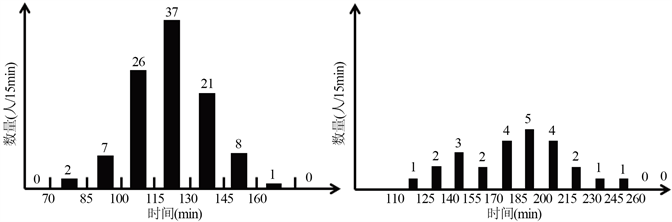Figure 1. Histogram of flows for 18 km and 31 km (cited from  )

$t=\frac{x}{v}$ (3)

2. 比赛数据的统计分析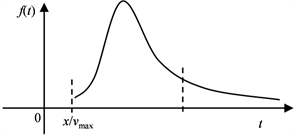Figure 2. Diagrammatic sketch of the probability density function $f\left( t \right)$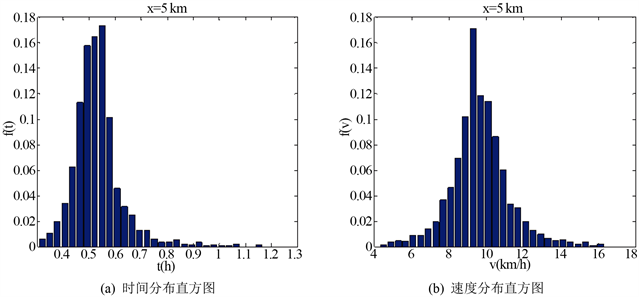Figure 3. Frequency histogram for x = 5 km water station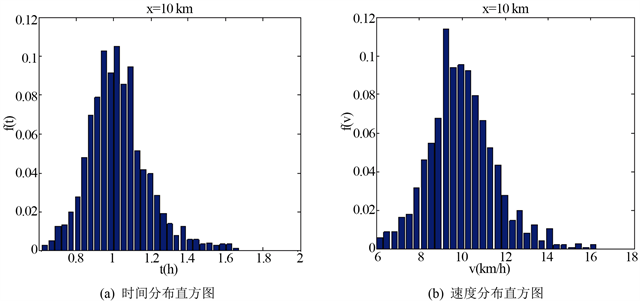Figure 4. Frequency histogram for x = 10 km water station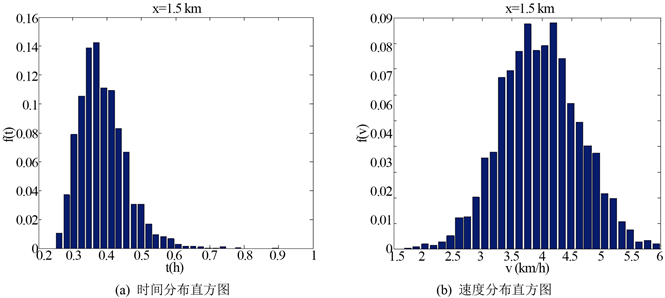Figure 5. Frequency histogram of the swimming results

3. 速度的概率密度函数

$f\left(v\right)=\frac{1}{\sigma \sqrt{2\text{π}}}\mathrm{exp}\left[-\frac{{\left(v-\mu \right)}^{2}}{2{\sigma }^{2}}\right]=N\left(\mu ,{\sigma }^{2}\right)$ (4)

$\mu =\stackrel{¯}{v}=\frac{{v}_{1}+{v}_{2}+,\cdots ,+{v}_{K}}{K}$ (5)

$\sigma =std\left(v\right)=\sqrt{\frac{{\left({v}_{1}-\stackrel{¯}{v}\right)}^{2}+{\left({v}_{2}-\stackrel{¯}{v}\right)}^{2}+,\cdots ,+{\left({v}_{K}-\stackrel{¯}{v}\right)}^{2}}{K-1}}$ (6)

4. 时间的概率密度函数

${f}_{Y}\left(y\right)=\left\{\begin{array}{cc}{f}_{X}\left[h\left(y\right)\right]|{h}^{\prime }\left(y\right)|,& y\in \left(a,b\right)\\ 0,\begin{array}{cccc}& & & \end{array}& 其他\end{array}$ (7)

$f\left(t\right)=\frac{x/\left(\mu t\right)}{\left(t\sigma /\mu \right)\sqrt{2\text{π}}}\mathrm{exp}\left[-\frac{{\left(t-x/\mu \right)}^{2}}{2{\left(t\sigma /\mu \right)}^{2}}\right]=x/\left(\mu t\right)\cdot N\left(x/\mu ,{\left(t\sigma /\mu \right)}^{2}\right)$ (8)

5. 结论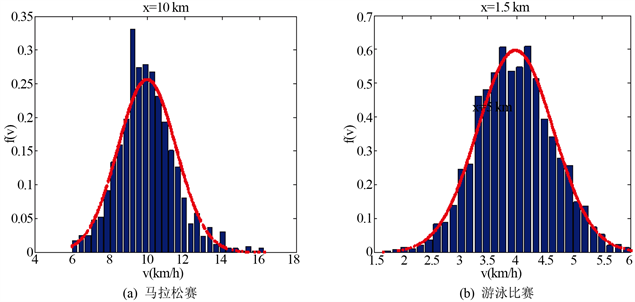Figure 6. Comparison of normal probability density function and frequency histogram of speed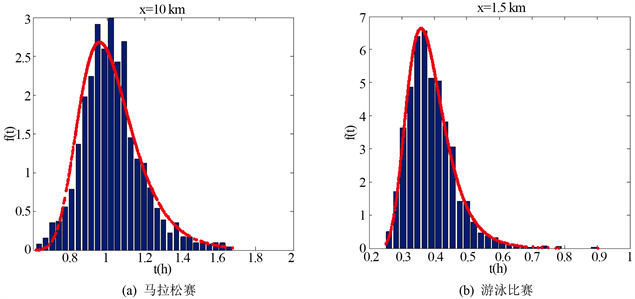Figure 7. Comparison of normal-like probability density function and frequency histogram of time

 田利军. 探析正态分布理论在体育运动统计分析中的作用[J]. 吉林师范大学学报(自然科学版), 2012(3): 148-151.

 许莉, 黄宗文. 体育统计常用指标的概率分布初探[J]. 体育科技, 1999, 20(2): 53-56.

 李栋, 杨明进. 马拉松服务方案优化研究[J]. 体育科学, 2008, 28(4): 30-38.

 中国田径协会官方网站. 2013重庆国际马拉松赛成绩表[EB/OL].
http://www.athletics.org.cn/competition/results/2013-07-14/414937.html

 Consortium for Mathematics and Its Applications (COMAP). HiMCM 2016 Problems.
http://www.comap.com/highschool/contests/himcm/2016problems.html

 茆诗松, 程依明, 濮晓龙. 概率论与数理统计教程[M]. 北京: 高等教育出版社, 2014.

Top